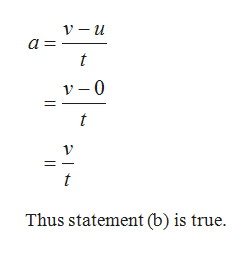# A racing car starts from rest and reaches a final speed v in a time interval of tafter traveling a distance on a straight line. If the acceleration a of the car is constantduring this time, which of the following statements can not be true? (a) The car travelsa distance of v2/2at. (b) The acceleration of the car is v/t. (c) The velocity of the car at t/2(at one half of the time interval t) is 1/2 at2.A) Statement (a) onlyB) Statement (b) onlyC) Statement (a) and (c)D) Statements (b) and (c)

Question
29 views

A racing car starts from rest and reaches a final speed v in a time interval of t
after traveling a distance on a straight line. If the acceleration a of the car is constant
during this time, which of the following statements can not be true? (a) The car travels
a distance of v2/2at. (b) The acceleration of the car is v/t. (c) The velocity of the car at t/2
(at one half of the time interval t) is 1/2 at2.
A) Statement (a) only
B) Statement (b) only
C) Statement (a) and (c)
D) Statements (b) and (c)

check_circle

star
star
star
star
star
1 Rating
Step 1

From Newton’s equation of motion

Step 2

The acceleration i...help_outlineImage TranscriptioncloseV — и а - v - 0 t t Thus statement (b) is true. fullscreen

### Want to see the full answer?

See Solution

#### Want to see this answer and more?

Solutions are written by subject experts who are available 24/7. Questions are typically answered within 1 hour.*

See Solution
*Response times may vary by subject and question.
Tagged in

### Physics